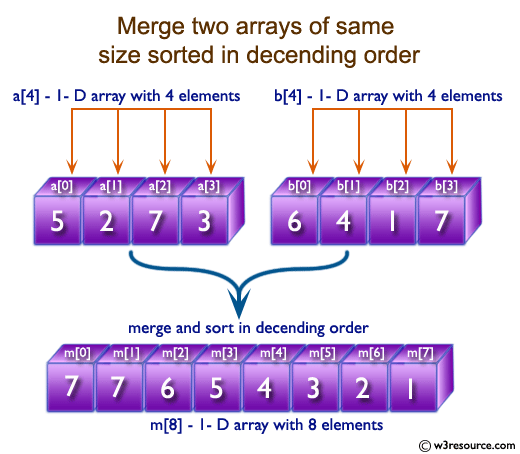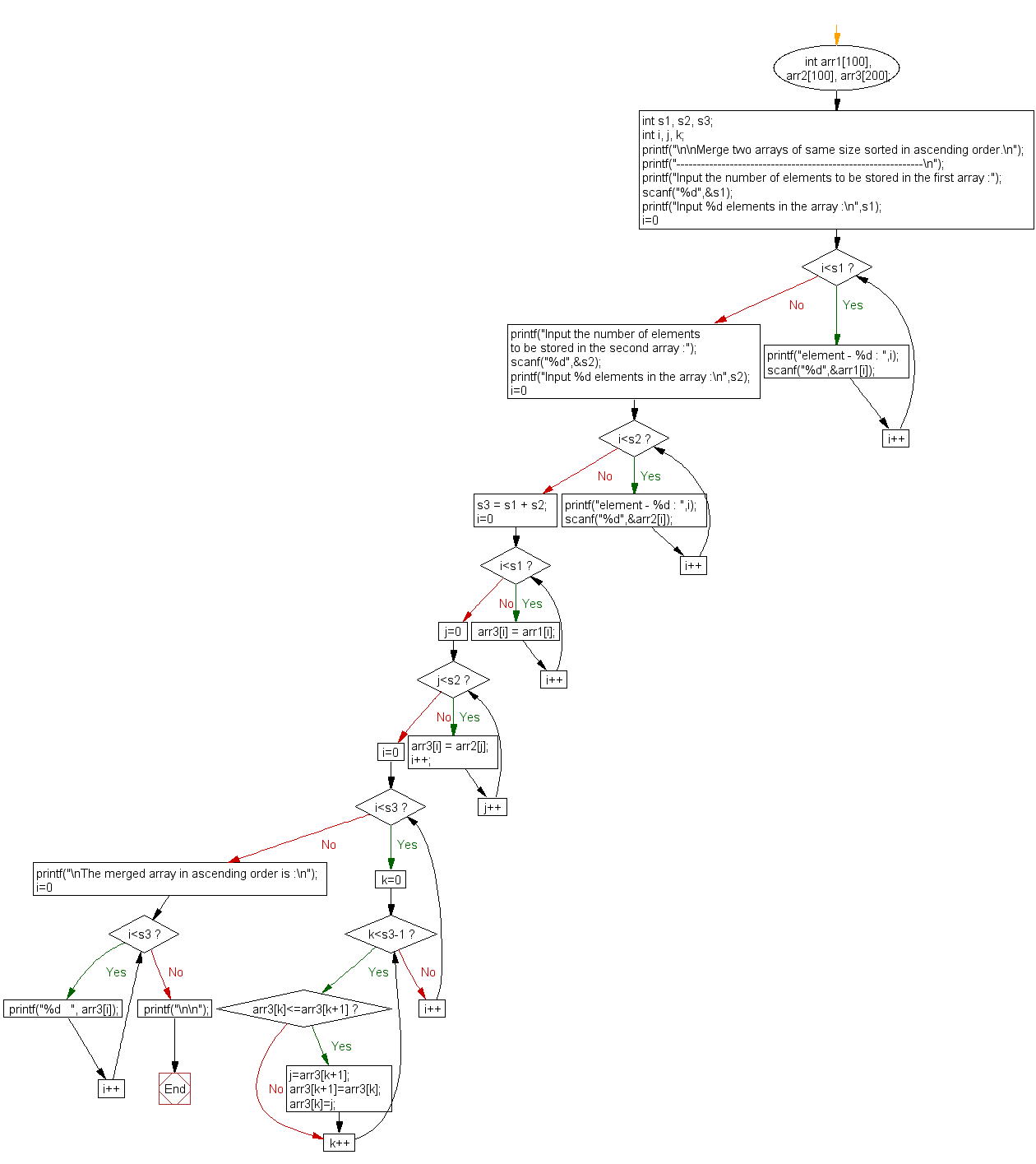﻿ C exercises: Merge two arrays of same size sorted in decending order - w3resource# C Exercises: Merge two arrays of same size sorted in decending order

## C Array: Exercise-7 with Solution

Write a program in C to merge two arrays of same size sorted in decending order.

Pictorial Presentation:Sample Solution:

C Code:

``````#include <stdio.h>

void main()
{
int arr1, arr2, arr3;
int s1, s2, s3;
int i, j, k;

printf("\n\nMerge two arrays of same size sorted in decending order.\n");
printf("------------------------------------------------------------\n");

printf("Input the number of elements to be stored in the first array :");
scanf("%d",&s1);

printf("Input %d elements in the array :\n",s1);
for(i=0;i<s1;i++)
{
printf("element - %d : ",i);
scanf("%d",&arr1[i]);
}

printf("Input the number of elements to be stored in the second array :");
scanf("%d",&s2);

printf("Input %d elements in the array :\n",s2);
for(i=0;i<s2;i++)
{
printf("element - %d : ",i);
scanf("%d",&arr2[i]);
}

/* size of merged array is size of first array and  size of second array */
s3 = s1 + s2;
/*----------------- insert in the third array------------------------------------*/
for(i=0;i<s1; i++)
{
arr3[i] = arr1[i];
}
for(j=0;j<s2; j++)
{
arr3[i] = arr2[j];
i++;
}
/*----------------- sort the array in decending order ---------------------------*/
for(i=0;i<s3; i++)
{
for(k=0;k<s3-1;k++)
{

if(arr3[k]<=arr3[k+1])
{
j=arr3[k+1];
arr3[k+1]=arr3[k];
arr3[k]=j;
}
}
}

/*--------------- Prints the merged array ------------------------------------*/
printf("\nThe merged array in decending order is :\n");
for(i=0; i<s3; i++)
{
printf("%d   ", arr3[i]);
}
printf("\n\n");
}
```
```

Sample Output:

```Merge two arrays of same size sorted in decending order.
------------------------------------------------------------
Input the number of elements to be stored in the first array :3
Input 3 elements in the array :
element - 0 : 1
element - 1 : 2
element - 2 : 3
Input the number of elements to be stored in the second array :3
Input 3 elements in the array :
element - 0 : 1
element - 1 : 2
element - 2 : 3

The merged array in decending order is :
3   3   2   2   1   1
```

Flowchart:C Programming Code Editor:

Improve this sample solution and post your code through Disqus.

What is the difficulty level of this exercise?

﻿

## C Programming: Tips of the Day

Where is the C auto keyword used?

auto is a modifier like static. It defines the storage class of a variable. However, since the default for local variables is auto, you don't normally need to manually specify it.

Ref : https://bit.ly/3yzwC9r